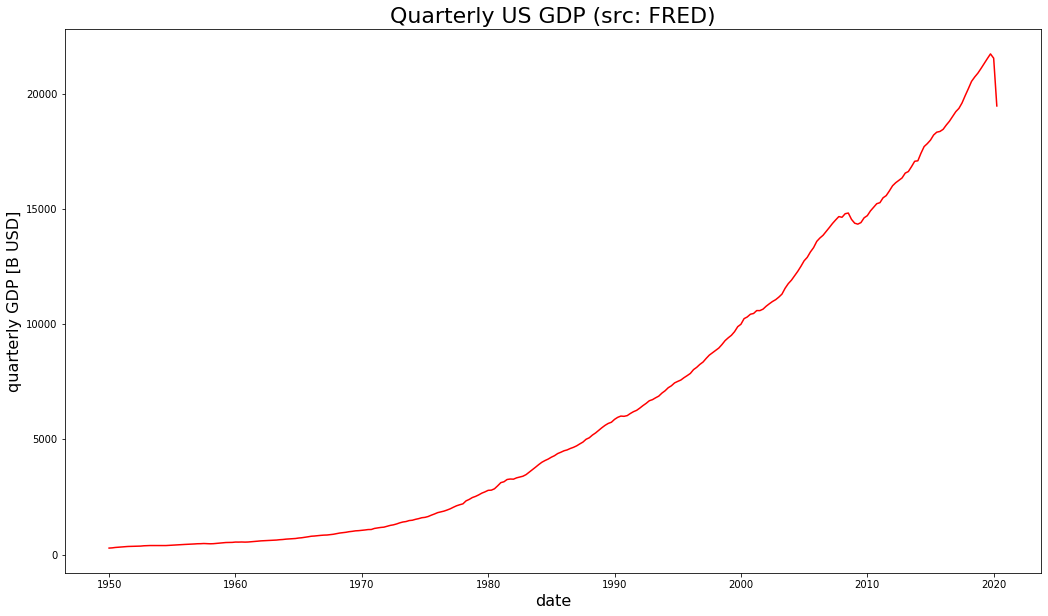# Pandas datareader for FRED quarterly GDP data queries in PythonIn other posts I have demonstrated how to use the quandl module in Python for retrieving equity or stock prices. I also showed how to query stock prices from Yahoo, using pandas_datareader in Python. I also demonstrated OECD and FRED interfaces in R and Python, in the form of packages and modules.

In this post I will demonstrate how to query quarterly GDP time series data from FRED – using pandas_datareader in Python.

In below lines of code I import relevant modules and query quarterly GDP data from FRED:

```# import relevant modules
import pandas_datareader.data as web
import datetime
# define datetimes for start and end dates
start_date = datetime.datetime(1950, 1, 1)
end_date = datetime.datetime(2020, 9, 29)
# import stock data for given period between start and end date form yahoo finance
df = web.DataReader("GDP","fred",start_date,end_date)
# display head of dataframe
df.head()
```

Using matplotlib.pyplot I can visualize the development in quarterly GDP over time. This is what I do in the lines of code below:

```# import matplotlib.pyplot
import matplotlib.pyplot as plt
# create figure
plt.figure(figsize=(17.5,10))
# create line plot for closing prices
plt.plot(df.index,df["GDP"],color="red")
# add title to plot
plt.title("Quarterly US GDP (src: FRED)",size=22)
# add x-axis label
plt.xlabel("date",size=16)
# add y-axis label
plt.ylabel("quarterly GDP [B USD]",size=16)
```
`Text(0, 0.5, 'quarterly GDP [B USD]')`

### Leave a Reply

This site uses Akismet to reduce spam. Learn how your comment data is processed.